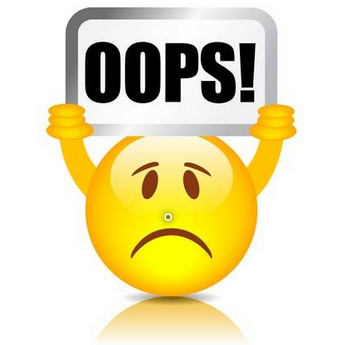Array (  =>  => questions  => Basic  => Decimal-to-Binary- ) Basic | Decimal to Binary | THE INQUISITIVE

### Decimal to Binary

#### Description

Given a integer N. You have to convert the given number into binary number.

#### Input Format

First line contains a integer N.

#### Output Format

Print the binary number for the given N.

Example 1:

Input
`5`
Output
`101`
Example 2:

Input
`10`
Output
`1010`
Example 3:

Input
`16`
Output
`10000`### Approach

Approach 1: Using mod 2 operation

Step-i) Initialize a string to empty string
Step-ii) get the remainder by dividing the number with 2
Step-iii) append remainder to the string
Step-iv) divide number by 2
Step-v) Repeat ii, iii and iv till the number is greater than zero
Step-vi) return the string

Time Complexity: O(logn)
Space Complexity: O(logn) as the final string is of size logn

Approach 2: Using builtin function

Step-i) Call the built in function Integer.toBinaryString() and pass number as parameter
Step-ii) Return result of the above built in function

Time Complexity: O(logn)
Space Compleixty : O(logn)

Appraoch 3: Using Array

Step-i) Take a array of sufficient size (here for explanation it is taken as 20)
Step-ii) Divide the number by 2 and assign the remainder to array
Step-iii) Increase the index and divide the number by 2
Step-iv)Repeat the steps ii and iii till the number is greater than 0
Step-v) Take an empty string
Step-vi) From current index of the array append current element to the string
Step-vii) Decrease the index
Step-viii) Repeat steps vi and vii till index is greater than or equal to 0
Step-ix) Return the string

Time Complexity: O(logn)
Space Complexity: O(logn) as the array size is constant as created only string grows to the size of logn to represent the binary number

Note :

Let us know if you can come up with a better approach, mail us at support@theinquisitive.in Your approach will be reviewed and posted with credits to you.